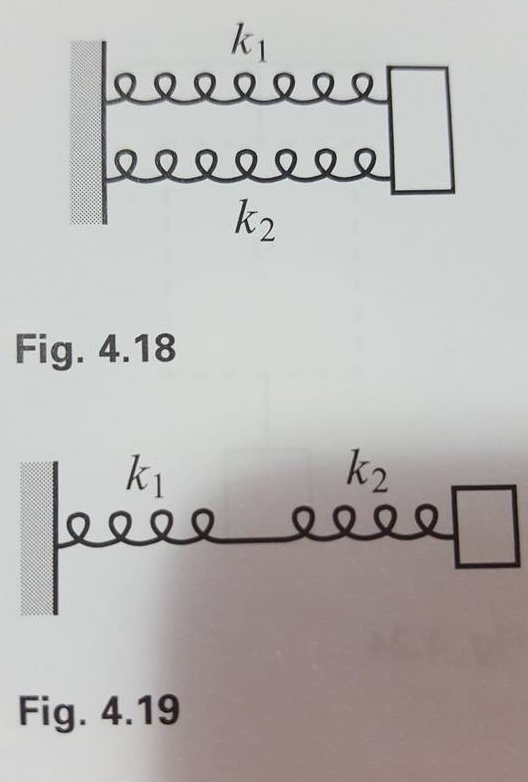# Problem: Two springs with spring constants k1 and k2 are connected in parallel, as shown in Fig. 4.18. What is the effective spring constant, keff? In other words, if the mass is displaced by x, find the keff for which the force equals F = -keffx.

###### FREE Expert Solution

Hooke's law:

$\overline{){\mathbf{F}}{\mathbf{=}}{\mathbf{k}}{\mathbf{·}}{\mathbf{∆}}{\mathbf{x}}}$

Δx is the same for parallel springs.

Δx = Δx1 = Δx2

88% (366 ratings)###### Problem Details

Two springs with spring constants kand k2 are connected in parallel, as shown in Fig. 4.18. What is the effective spring constant, keff? In other words, if the mass is displaced by x, find the keff for which the force equals F = -keffx.